# Finding an equation of best fit in Desmos

//Finding an equation of best fit in Desmos

## Finding an equation of best fit in Desmos

1. Go to Desmos.com and choose Start Graphing.
2. In the upper left, choose Add Item > table.
3. Type your data in the table.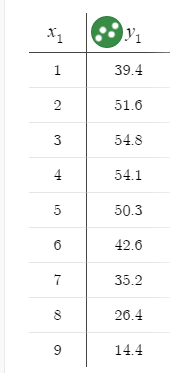4. Click on the wrench in the upper right to change the graph settings.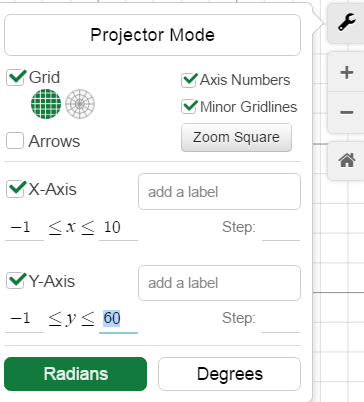6. Decide what type of equation the data represents. In the example above, the data appears to be quadratic.
7. In the input area, type y=a(x-h)^2 + k and press Enter. Sliders will be added for a, h, and k.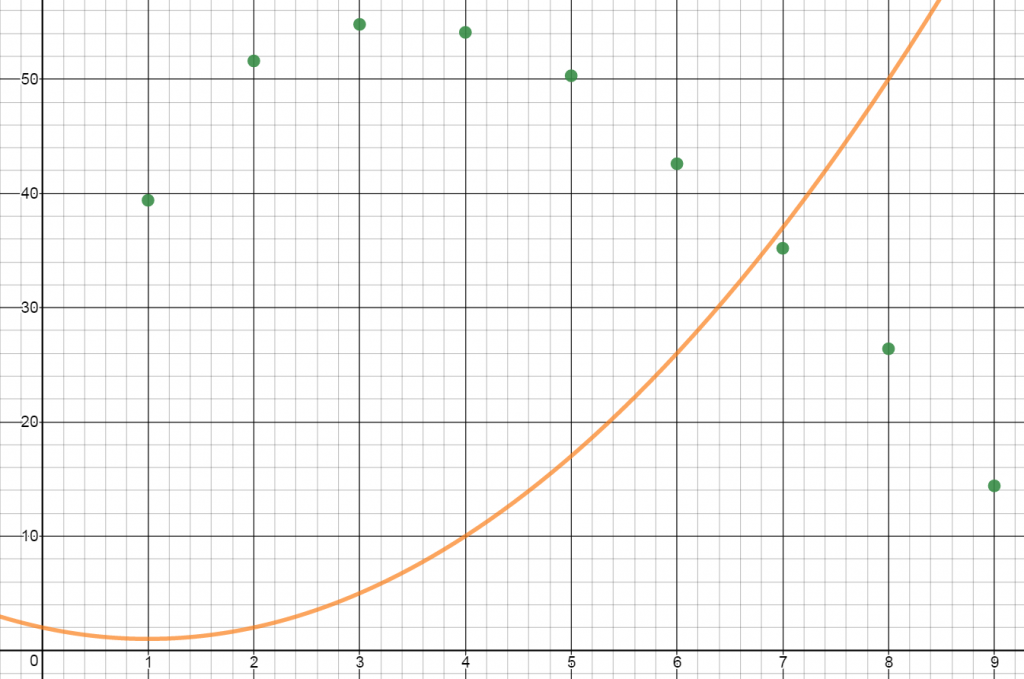8. Adjust the values of the sliders until the graph of the equation most closely fits your data points. You will likely need to change your slider settings. To do so, click on the gear icon to Edit List.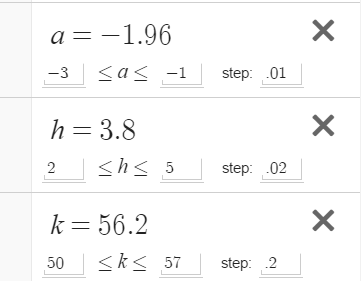9. The R-squared value is a statistical measure of how close the data are to a fitted regression line. The closer R2 is to 1, the better the curve matches the data. To have Desmos calculate your R2 value in a new input line type y1 ~ a(x1-h)^2+k. Desmos uses y1 to represent the y-value in a data table and x1 to represent the x-values in a table.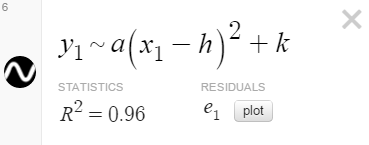10. Adjust your sliders until you get the highest possible value for R². Write down your equation of best fit.
11. To have Desmos create an equation of best fit, in the input bar, add a new equation y1~bx1^2+cx1+d. Compare the equation Desmos generated to yours.
By | 2017-11-13T22:01:55+00:00 September 24th, 2016|Uncategorized|2 Comments

### About the Author: Stacie Bender1.2.